Introduction to Analysis : Integrability of Real Numbers (R)

Example Questions

Example Question #1 : Riemann Integral, Riemann Sums, & Improper Riemann Integration

What conditions are necessary to prove that the upper and lower integrals of a bounded function exist?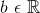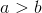,  and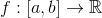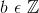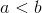,  andbe bounded,  andbe bounded,  and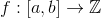be bounded,  and,  andbe bounded

Explanation:

Using the definition for Riemann sums to define the upper and lower integrals of a function answers the question.

According the the Riemann sum where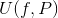represents the upper integral and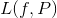the following are defined:

1. The upper integral ofon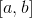is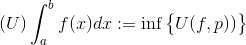whereis a partition of.

2. The lower integral ofonis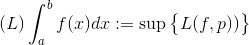whereis a partition of.

3. If 1 and 2 are the same then the integral is said to be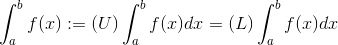if and only if,  andbe bounded.

Therefore the necessary condition for the proving the upper and lower integrals of a bounded function exists is if and only if,  andbe bounded.

Example Question #2 : Riemann Integral, Riemann Sums, & Improper Riemann Integration

What term has the following definition.and. Over the intervalis a set of points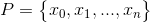such that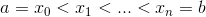Partition

Upper Riemann sum

Norm

Refinement of a partition

Lower Riemann sum

Partition

Explanation:

By definition

Ifand.

A partition over the intervalis a set of pointssuch that.

Therefore, the term that describes this statement is partition.

Example Question #3 : Riemann Integral, Riemann Sums, & Improper Riemann Integration

What term has the following definition.

The __________ of a partitionis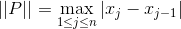Norm

Refinement of a partition

Lower Riemann sum

Partition

Upper Riemann Sum

Norm

Explanation:

By definition

Ifand.

A partition over the intervalis a set of pointssuch that.

Furthermore,

The norm of the partitionisTherefore, the term that describes this statement is norm.